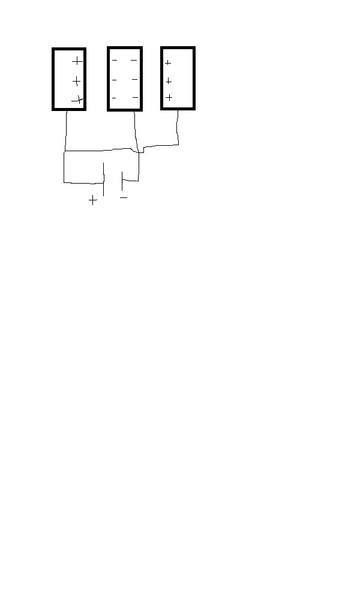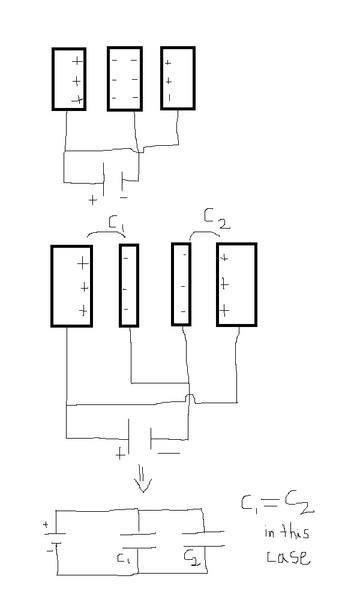# Variable Air Filled Capacitor

## Homework Statement

A variable air-filled capacitor of the type used in tuning radios
is shown. Alternate plates are connected together, one group
fixed in position (shown in dark grey), the other group capable
of rotation (shown in light gray). Consider a pile of n plates of
alternate polarity, each having an area A and separated from
adjacent plates by a distance d.

Show that this capacitor has a maximum capacitance of:
C=((n-1)ε0A)/d

Picture for reference

2. The attempt at a solution

I'm pretty sure I am correct, but something about my approach seems.. too simple. My idea is that to find the capacitance you must simply add up the capacitance between every plate. Since if there n plates, there are n-1 separations and thus C=((n-1)ε0A)/d.

I can't logically find another way to calculate the capacitance, but it can't possibly be that easy?

Seems right to me. It would be more complicated if they asked you to form an equation giving the capacitance based on an angle of rotation (and therefore variable overlap), but beyond that, I believe this is the correct approach.

Thanks, I was also wondering why is it that its wrong to model the capacitor as many capacitors in series. So for example, the 8 plate capacitor in the picture could be modeled as four two-plate capacitors in series. Why is it that I should add the capacitances as if they were in parallel? Am I missing some fundamental concept?

If you notice, there are two rods: one connects to one set of plates (the light ones), and the second rod to the other (the dark ones). As these represent polarities, thus you can see that all the negative terminals and all the positive terminals are linked together, thus they're in parallel.

So in this case, since they are in parallel as you say, Ceq=((n-1)ε0A)/d. But if they were in series I would say:

1/Ceq = (n-1)(1/((ε0A)/d)) which equals
Ceq = (ε0A)/(d(n-1))

And they would only be in series if all the plates are connected only by one rod? Also, I don't quite understand your explanation of why they are in parallel, if you could please explain in more detail I would greatly appreciate it

Thanks for all the help

Last edited:
basically i think the idea is how to calculate the capacitance when the plates are lined up side by side and voltage + and - ends are then connected to the plates alternately starting from one side lets say left . now lets consider 3 plates connected like that, what happens , are they in series or in parallel ,in the above picture there are three such plates and they are connected to + and - terminals alternately , now we should remember that since the plates are of same size and spaced evenly then the surface charge accumulation will be equal on all the plates since they have equal capacitance which depends only on dimensions of plate and the distance between them .Since charge can neither be created nor destroyed, we are assuming here that three + charges are induced by the +terminal on left plate , now the middle plate will accumulate surface charges on both sides because middle plate is contributing to the capacitance on both sides and the charge on middle plate is three - charges on both sides . if we look at picture carefully we can see that there is no extra charge created by the potential difference applied by the battery , i mean in total there are six + charges and six - charges which is expected . now lets divide the middle in two parts and connect both to - terminal of the battery this action will not affect the capacitance neither it will create extra charges on the plates ( i mean it will not induce four or five - charges on the two separate middle plates) . Picture below is self explanatory and is for the case when all the plates are of same area A and distance d since C= epsilon (0) A/ dthis explains that the three plates lined up side by side and charged aternately positive and negative are in parallel and the configuration has two capacitors in parallel , extending this to n plates we get (n-1) capacitors connected in parallel.

thanks

Last edited: